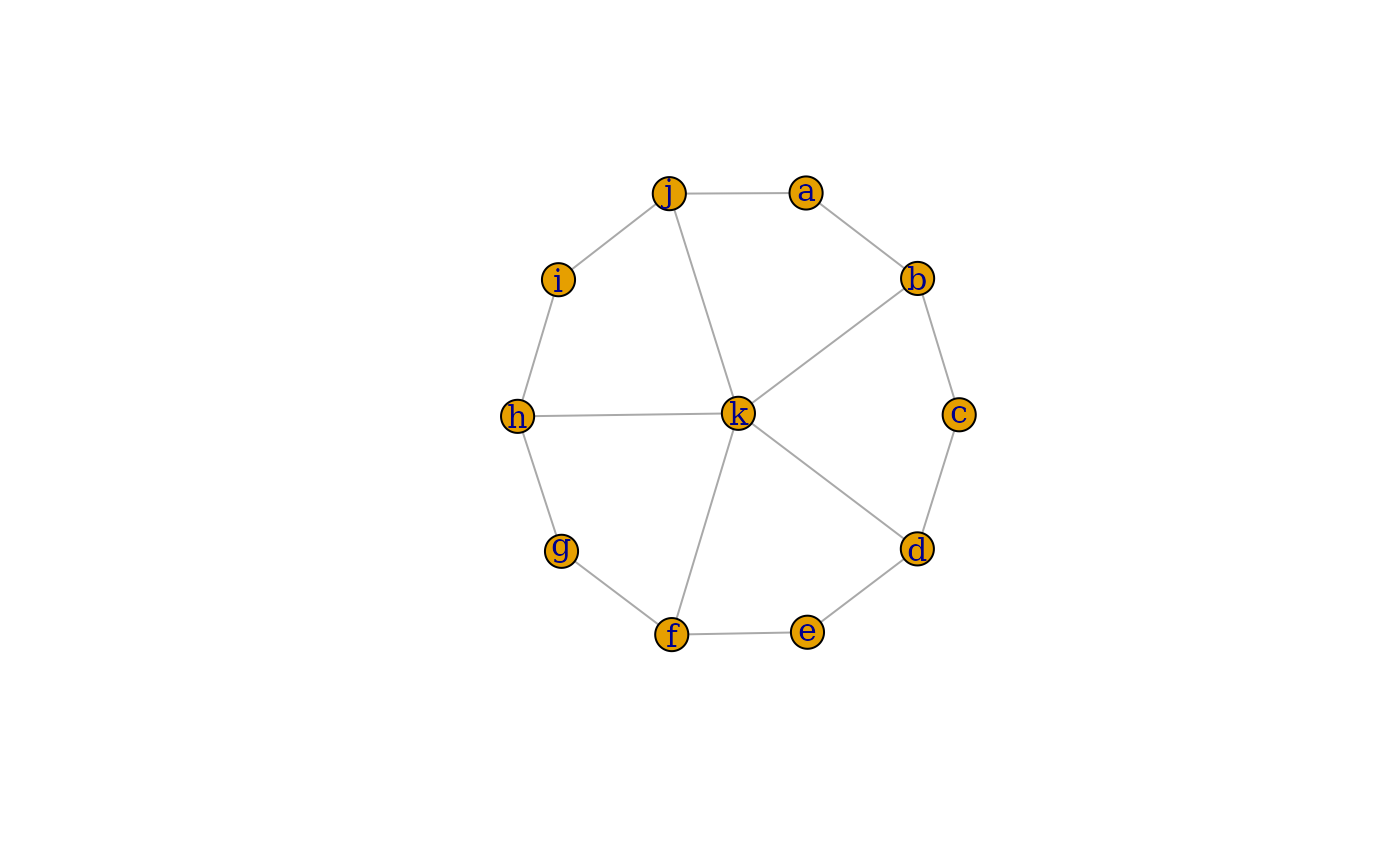The difference of two graphs are created.

## Usage

# S3 method for igraph
difference(big, small, byname = "auto", ...)

## Arguments

big

The left hand side argument of the minus operator. A directed or undirected graph.

small

The right hand side argument of the minus operator. A directed ot undirected graph.

byname

A logical scalar, or the character scalar auto. Whether to perform the operation based on symbolic vertex names. If it is auto, that means TRUE if both graphs are named and FALSE otherwise. A warning is generated if auto and one graph, but not both graphs are named.

...

Ignored, included for S3 compatibility.

## Value

A new graph object.

## Details

difference() creates the difference of two graphs. Only edges present in the first graph but not in the second will be be included in the new graph. The corresponding operator is %m%.

If the byname argument is TRUE (or auto and the graphs are all named), then the operation is performed based on symbolic vertex names. Otherwise numeric vertex ids are used.

difference() keeps all attributes (graph, vertex and edge) of the first graph.

Note that big and small must both be directed or both be undirected, otherwise an error message is given.

Other functions for manipulating graph structure: +.igraph(), add_edges(), add_vertices(), complementer(), compose(), connect(), contract(), delete_edges(), delete_vertices(), difference(), disjoint_union(), edge(), igraph-minus, intersection.igraph(), intersection(), path(), permute(), rep.igraph(), reverse_edges(), simplify(), union.igraph(), union(), vertex()

## Author

Gabor Csardi csardi.gabor@gmail.com

## Examples


## Create a wheel graph
wheel <- union(
make_ring(10),
make_star(11, center = 11, mode = "undirected")
)
V(wheel)$name <- letters[seq_len(vcount(wheel))] ## Subtract a star graph from it sstar <- make_star(6, center = 6, mode = "undirected") V(sstar)$name <- letters[c(1, 3, 5, 7, 9, 11)]
G <- wheel %m% sstar
print_all(G)
#> IGRAPH e5cfa2f UN-- 11 15 --
#> + attr: name_1 (g/c), name_2 (g/c), mutual (g/l), circular (g/l), mode
#> | (g/c), center (g/n), name (v/c)
#> + edges (vertex names):
#> a -- b, j
#> b -- a, c, k
#> c -- b, d
#> d -- c, e, k
#> e -- d, f
#> f -- e, g, k
#> g -- f, h
#> h -- g, i, k
#> i -- h, j
#> j -- a, i, k
#> k -- b, d, f, h, j
plot(G, layout = layout_nicely(wheel))